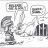# Fibline Glance: Bollinger Bands and EMA Indicator for ThinkorSwim#### BenTen

Staff
VIP
Warehouse
This is an indicator from TradingView called Fibline Glance that combines two Bollinger Bands and an exponential moving average. According to the author, it was based on "a set of three indicators combined into one script". The source of the three indicators come from another TV user (fibline).

To me, the orange line does an excellent job of showing support and resistance
The orange line is now a yellow line in the ThinkorSwim version.### thinkScript Code

Rich (BB code):
``````# WalkingBallista & BenTen
# https://usethinkscript.com/d/102-fibline-glance-bollinger-bands-and-ema-indicator-for-thinkorswim

# Fast Cloud
input price = close;
input displace = 0;
input length = 9;
input Num_Dev_Dn = -0.1;
input Num_Dev_up = 0.1;
input averageType = AverageType.Simple;

def sDev = stdev(data = price[-displace], length = length);

def MidLine = MovingAverage(averageType, data = price[-displace], length = length);
plot LowerBand = MidLine + num_Dev_Dn * sDev;
plot UpperBand = MidLine + num_Dev_Up * sDev;

# Slow Cloud
input slow_price = close;
input slow_displace = 0;
input slow_length = 40;
input slow_Num_Dev_Dn = -0.4;
input slow_Num_Dev_up = 0.4;
input slow_averageType = AverageType.Simple;

def slow_sDev = stdev(data = slow_price[-slow_displace], length = slow_length);

def slow_MidLine = MovingAverage(averageType, data = slow_price[-slow_displace], length = slow_length);
plot slow_LowerBand = slow_MidLine + slow_num_Dev_Dn * slow_sDev;
plot slow_UpperBand = slow_MidLine + slow_num_Dev_Up * slow_sDev;

LowerBand.SetDefaultColor(GetColor(2));
UpperBand.SetDefaultColor(GetColor(2));

slow_LowerBand.SetDefaultColor(GetColor(1));
slow_UpperBand.SetDefaultColor(GetColor(1));

DefineGlobalColor("Fast_Cloud", Color.Orange);
DefineGlobalColor("Slow_Cloud", Color.Cyan);Indicators 17